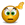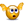# Sortino ratio

## Excel Help for Sortino Ratio in Analyzing Corporate Financial DataHello and thank you for both the template and the ability to post qusetions.  My question pertains to the calculation of the Sortino Ratio.  As per Dr. Frank Sortino the ratio is the portfolio return minus the target rate of return over the downside risk or semi variation ( http://en.wikipedia.org/wiki/Sortino_ratio)  I have chose to optimize a sample portfolio with Semi-Deviation as my risk, with maximum Sortino as the result.  I ran the optimization twice and only changed the risk free rate... but the Sortino ratio for the portfolios changed.  How come?  Secondly the optimizer allocated a large portion of the portfolio to an asset whose estimate return is below the target, but it is still showing a positive Sortino ratio.  How does that happen?Again thank you for you assistance. M. Tilden Posted by mtilden on 15 Nov 2013Rate this: (4.3/5 from 3 votes) When using the semi downside deviation under the Sortino ratio the current portfolio ration will not change between optimizations.  If, however the weightings in subsequent optimizations changes, then logically the resulting Sortino ratio will also change. The ratio will also change when changes are made to the risk free rate as this is used by deducting from return in the numerator of the ratio.A large weighting for an asset can be made even though the return is low as its contribution to overall risk may be significant as either low risk or negatively correlated to other assets risk. Excel Business Forums Administrator Posted by Excel Helper on 15 Nov 2013Rate this: (3/5 from 1 vote) Thank you for your reply.  So the Sortino Ratio is using the risk free rate instead of the target rate in the calculation? M. Tilden Posted by mtilden on 15 Nov 2013Rate this: (3/5 from 1 vote) Yes.  The Sortino ratio is implemented to use the risk free rate in the numerator deducted from return and the target rate used in the downside deviation of the denominator.  To make the Sortino ratio calculate with only the target rate, we can equate the risk free rate to the target return rate. Excel Business Forums Administrator Posted by Excel Helper on 15 Nov 2013